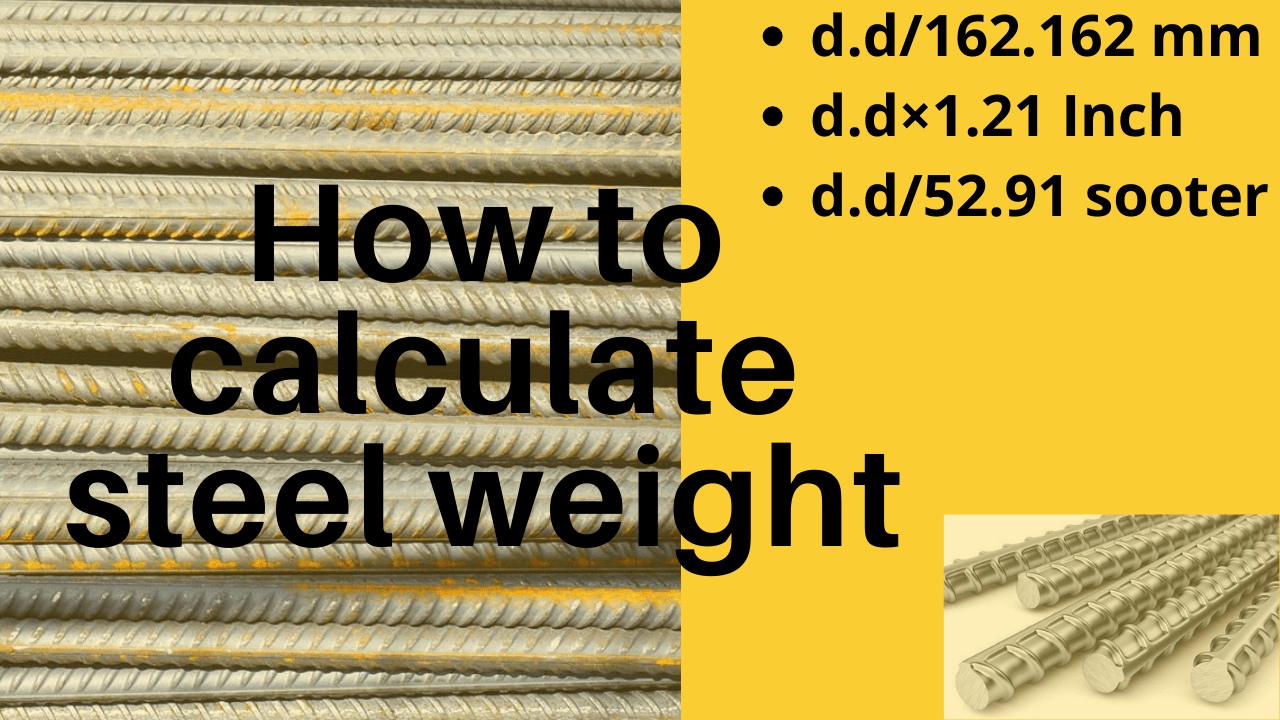# How to steel bar weight calculate?

Steel or reinforcement is an important element in civil engineering & construction field. If you are to to know How to do steel weight calculation or want to know the steel calculation formula. You are on right blog.

Steel is being used in construction for a long time. Steel got importance because of its tensile strength. As we know concrete is good in compressive strength but poor in tension, So the steel bars, reinforcement, wire meshes are being used in construction of structures with concrete.

## Calculation of steel weight in meters (by dia in mm):

How do you calculate the weight of steel? Its really simple to calculate weight of steel in meter/kg by diameter in millimeter.

What is the formula of steel weight calculation? Formula for per unit weight of steel in meter = D2/162.

What is 162 in steel weight calculation? 162 is derived constant value that is used to find the per unit meter weight of steel.

Steel bar weight per meter calculation of 12mm dia steel?

1. Simply take square of diameter of steel bar in millimeter and divide by 162. It will give the Weight of per steel in meter.
2. Steel bar= 12mm dia
3. weight = (12)2 ÷ 162
4. w.t = 144÷162
5. weight of 12mm steel bar per meter= 0.888kg/m

with this formula you can get per meter weight of any steel bar. To get the total weight simply multiply 0.889 with total length of steel in meters.

Read also quantity of plaster material calculation.

## Steel bar weight per foot calculation (by dia in inches):

Formula for per unit weight of steel in foot = D2 x 1.21

1. Simply take square of diameter of steel bar in inches and multiply by 1.21. It will give the weight of steel in feet.
2. Steel bar = 0.5 inch (4 sooter)
3. w.t = (0.5)2 x 1.21
4. w.t = 0.25 x 1.21
5. Weight of 0.5 inch (4 sooter) bar per foot = 0.302kg/ft

Weight of 0.75 inch (6 sooter) bar per foot =0.752 × 1.21 = 0.680kg/ft

with this formula you can get per foot weight of any steel bar. To get the total weight simply multiply 0.302 with total length of steel in feet.

## Structural steel weight calculation (by bar no):

If you want to calculate per foot weight of steel bar in sooter or bar number. Following are the formula for that.

Formula for per unit weight of steel in foot = D2 ÷ 52.91

Steel bar = #3 , #4 , #6 , #8 (This is the number of steel bar or bar size in sooter)

Take the bar size in sooter or as a bar number.

1. Lets take #4 bar
2. Weight = 42 ÷ 52.91
3. weight of #4 steel bar per foot = 0.302 kg/ft
1. Lets take #6 bar
2. Weight = 62 ÷ 52.91
3. weight of #6 steel bar per foot = 0.680 kg/ft
Share